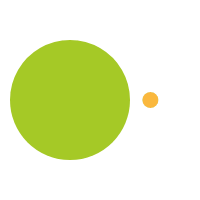# Salute!

February 28, 2013

Reversibility is an underlying concept in mathematical understanding. At a young age, this idea can manifest itself in the understanding that if there is a tall skinny glass of milk, you

can pour that milk into a short, wide glass and then back into the tall skinny glass and it’s original properties will be restored – in other words, the glass of milk will look just the same as when you started. As students advance, they will be able to apply this simple visual understanding to more complicated and abstract situations.

6 + 7 = 13 is the same thing as 7 + 6 = 13. And if that is true, then 13 – 7 = 6 and 13 – 6 = 7. This is also called the Commutative property, the idea that addends (numbers to be added) can be added in any order and the sum remains the same. Students will have an easier time grasping orders of operation, a crucial step in solving complex algebraic equations, if they have a strong foundation in the concept of reversibility.

Here is a game to practice Reversibility: